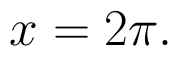Select Page

# CBSE Maths 12 Science Inverse Trigonometric Functions MCQ Answers in English

CBSE Maths 12 Science Inverse Trigonometric Functions MCQ Answers in English to enable students to get Answers in a narrative video format for the specific question.

Expert Teacher provides CBSE Maths 12 Science Inverse Trigonometric Functions MCQ Answers through Video Answers in English language. This video solution will be useful for students to understand how to write an answer in exam in order to score more marks. This teacher uses a narrative style for a question from Inverse Trigonometric Functions not only to explain the proper method of answering question, but deriving right answer too.

Please find the question below and view the Answer in a narrative video format.

Question:

## Similar Questions from CBSE, 12th Science, Maths, Inverse Trigonometric Functions

Question 1 : Using principal values, write the value of(View Answer Video)

Question 2 : Using principal values, write the value of(View Answer Video)

Question 3 :is equal to : (View Answer Video)

Question 4 : Ifthen find x. (View Answer Video)

Question 5 :

Write the value of the following:### Matrices

Question 1 : If, write the value of"x". (View Answer Video)

Question 2 : If, then find "a". (View Answer Video)

Question 3 : Find the value of x, if. (View Answer Video)

Question 4 : Find the value of z,  from the equation:(View Answer Video)

Question 5 : Compute:. (View Answer Video)

### Determinant

Question 1 : The following system of equations has x + 3y + 3z = 2, x + 4y + 3z = 1, x + 3y + 4z = 2,

Question 2 : Let, where. THhen (View Answer Video)

Question 4 : Evaluate the determinant:. (View Answer Video)

Question 5 :  Find the values of x, if. (View Answer Video)

### Application of Integrals

Question 1 : Find the area bounded by the curve y = sin x between x = 0 and(View Answer Video)

Question 2 : Find the area of the smaller part of the circlecut off by the line(View Answer Video)

Question 3 : Using the method of integration, find the area of the region bounded by the lines 3x - y - 3 = 0, 2x + y - 12 = 0 and x -2y - 1 = 0. (View Answer Video)

Question 4 : Using the method of integration, find the area of the region bounded by the lines: 5x - 2y -10 = 0, x + y - 9 = 0 and 2x - 5y - 4 = 0. (View Answer Video)

Question 5 : Find the area of smaller region bounded by the ellipseand the line. (View Answer Video)